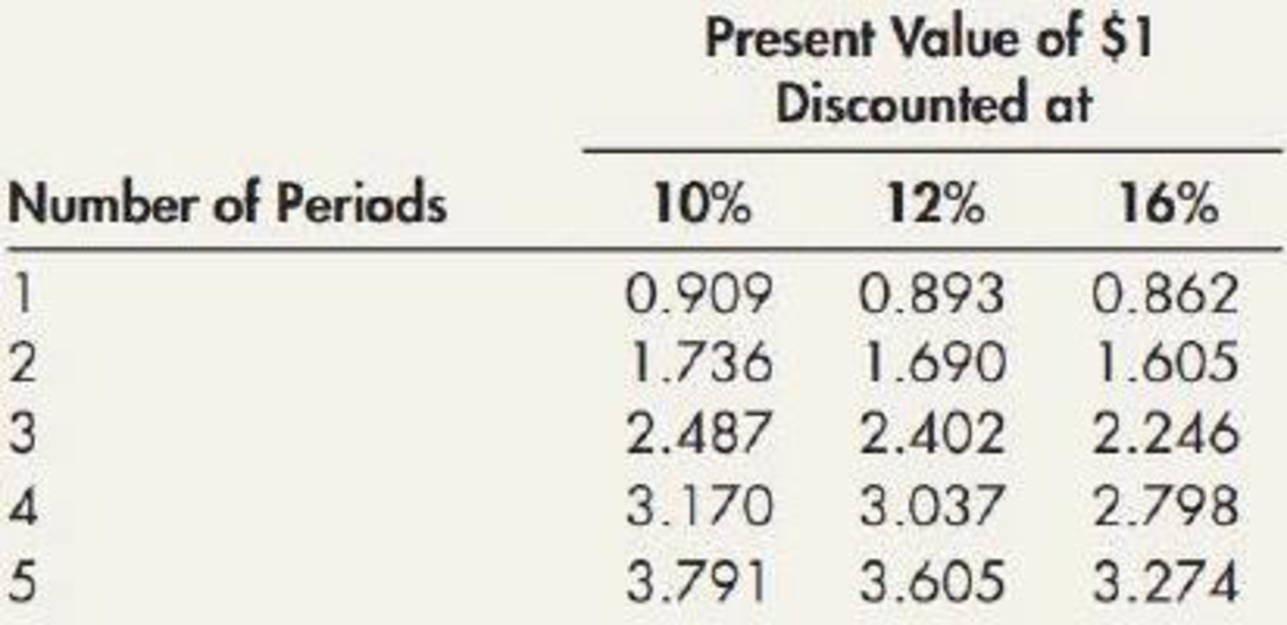Chapter M, Problem 6MC### Intermediate Accounting: Reporting...

3rd Edition
James M. Wahlen + 2 others
ISBN: 9781337788281

#### Solutions

Chapter
Section### Intermediate Accounting: Reporting...

3rd Edition
James M. Wahlen + 2 others
ISBN: 9781337788281
Textbook Problem
559 views

# An office equipment representative has a machine for sale or lease. If you buy the machine, the cost is $7,590. If you lease the machine, you will have to sign a noncancelable lease and make 5 payments of$2,000 each. The first payment will be paid on the first day of the lease. At the time of the last payment, you will receive title to the machine. The present value of an ordinary annuity of $1 is as follows:The interest rate implicit in this lease is approximately: a. 10% b. 12% c. between 10% and 12% d. 16% To determine Determine the approximate implicit interest rate of the lease. Explanation Interest: Interest is the cost of borrowing money, in other words Interest is the amount charged on the principal value of the money borrowed for the privilege of borrowing money. Interest is to be paid by the borrower and to be received by the lender. Given: Cost of buying the machine in single payment is$7,596.

Leasing requires 5 equal installment of $2,000, where first lease payment date is the first day of the lease. It is an annuity due, but only the present value of ordinary annuity table is provided for calculation. Determine rate of interest, if interest is compounded annually. PVD= Cash flow ×(pDn=5,i=?)$7,596= $2,000×(pOn-1=4,i=?-1)(pOn-1=4,i=?)=$7,596\$2,000-1(pOn-1=4,i=?)=3

### Still sussing out bartleby?

Check out a sample textbook solution.

See a sample solution

#### The Solution to Your Study Problems

Bartleby provides explanations to thousands of textbook problems written by our experts, many with advanced degrees!

Get Started